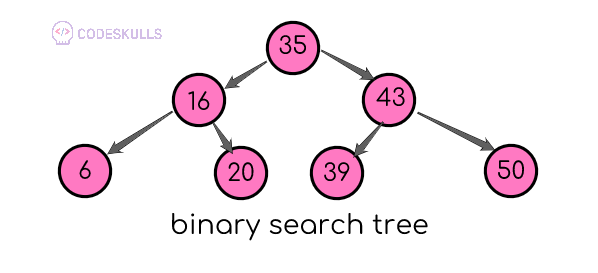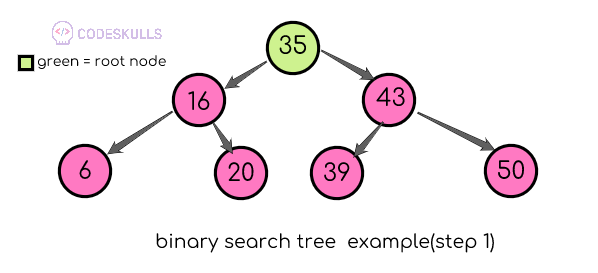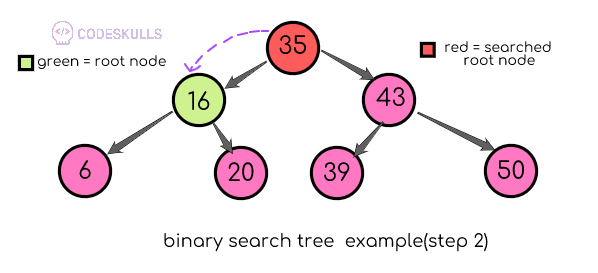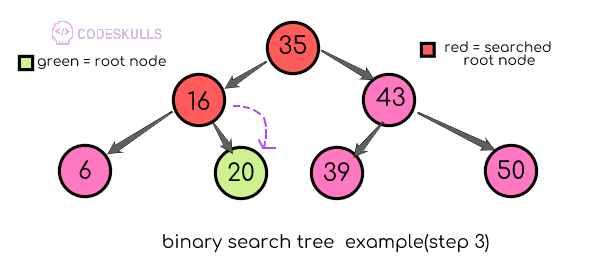# Searching a node in a binary search tree

• The search function is used to find whether the key element(value to find) is present in the binary tree or not
• the search always begins with the root node, key-value is compared with the root node
• if the value is greater than the root node, then search pointer moves to the right sub-tree
• if the value is less than the root node, then search pointer moves to the left sub-tree
• next subtree root node becomes the root node and the above process is done recursively on the present root node

let’s see one example, check whether number 20 is present in the below tree or notabove tree is a binary search because all elements in the left subtree is smaller is lesser than the root node 35 and all elements in the right dub tree are greater than the root nodestep 1:comapre 20 will root node 35, (20<35) 20 is less than 35 so the search will be continued on the left subtreestep 2: compare 20 will root node 16, (20>16) 20 is greater than 16 so the search will be continued on the right subtreestep 3: compare 20 will root node 20, (20==20) search node is equal to the root node, so the search node found

Last updated on by vishal devxo